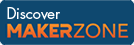Cody

# Problem 44342. Spot the First Occurrence of 5

Created by Peng Liu in Community

This problem series invites you to solve two simple problems related to the integer NUMBER FIVE, in order to celebrate Cody's 5th birthday.

In this problem, let's determine the position (index) of the first occurrence of 5 along the first non-singleton dimension of an input array.

E.g.,

• If the input is a vector, return the index of the first occurrence of 5.
```x = [1 2 5 3 5];
y = 3;
```
• If the input is a matrix, return the index of the first occurrence of 5 in each column. If 5 is not found, simply return 0;
```% Input x is a matrix
x = [1 2 5
5 9 1
5 6 5];
```
```% Output y
y = [2 0 1];
```

Next problem in this series: The Top 5 Primes

### Solution Stats

31.16% Correct | 68.84% Incorrect
Last solution submitted on Mar 20, 2019

#### TagsMATLAB and Simulink resources for Arduino, LEGO, and Raspberry Pi